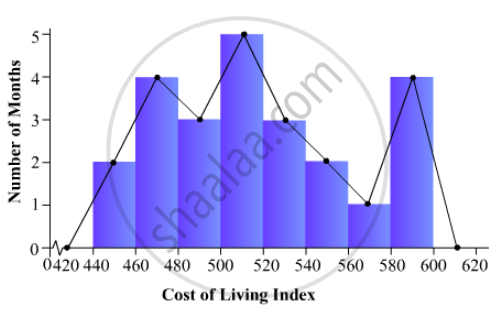# Draw, in the Same Diagram, a Histogram and a Frequency Polygon to Represent the Following Data Which Shows the Monthly Cost of Living Index of a City in a Period of 2 Years: - Mathematics

Draw, in the same diagram, a histogram and a frequency polygon to represent the following data which shows the monthly cost of living index of a city in a period of 2 years:

 Cost of livingindex: 440-460 460-480 480-500 500-520 520-540 540-560 560-580 580-600 No. of months: 2 4 3 5 3 2 1 4

#### Solution

To represent the given data by a histogram, we first draw horizontal and vertical axes. Let us consider that the horizontal and vertical axes represent the class-limits and the frequencies of the class-intervals respectively.

The given data is a continuous grouped frequency distribution with equal class-intervals. Construct rectangles with class-intervals as bases and respective frequencies as heights. It should be noted that the scale for horizontal axis may not be same as the scale for vertical axis. To draw the frequency polygon of the given data using histogram, obtain the mid-points of the upper horizontal side of each rectangle and then join these mid-points of the adjacent rectangles of the histogram by line segments. Obtain the mid-points of two class-intervals of 0 frequencies, i.e. on the horizontal axis, one adjacent to the first, on its left and one adjacent to the last, on its right. These class-intervals are known as imagined class-intervals. Complete the polygon by joining the mid-points of first and last class-intervals to the mid-points of imagined class-intervals adjacent to them. Let us take one vertical division is equal to 1 month.

The heights of the different rectangles are as follows:

1. The height of the rectangle corresponding to the class-interval 440-460 is 2 big divisions.

2. The height of the rectangle corresponding to the class-interval 460-480 is 4 big divisions.

3. The height of the rectangle corresponding to the class-interval 480-500 is 3 big divisions.

4. The height of the rectangle corresponding to the class-interval 500-520 is 5 big divisions.

5. The height of the rectangle corresponding to the class-interval 520-540 is 3 big divisions.

6. The height of the rectangle corresponding to the class-interval 540-560 is 2 big divisions.

7. The height of the rectangle corresponding to the class-interval 560-580is 1 big division.

8. The height of the rectangle corresponding to the class-interval 580-600 is 4 big divisions.

The histogram and frequency polygon of the given data is as follows:Concept: Graphical Representation of Data
Is there an error in this question or solution?

#### APPEARS IN

RD Sharma Mathematics for Class 9
Chapter 23 Graphical Representation of Statistical Data
Exercise 23.3 | Q 4 | Page 41

Share